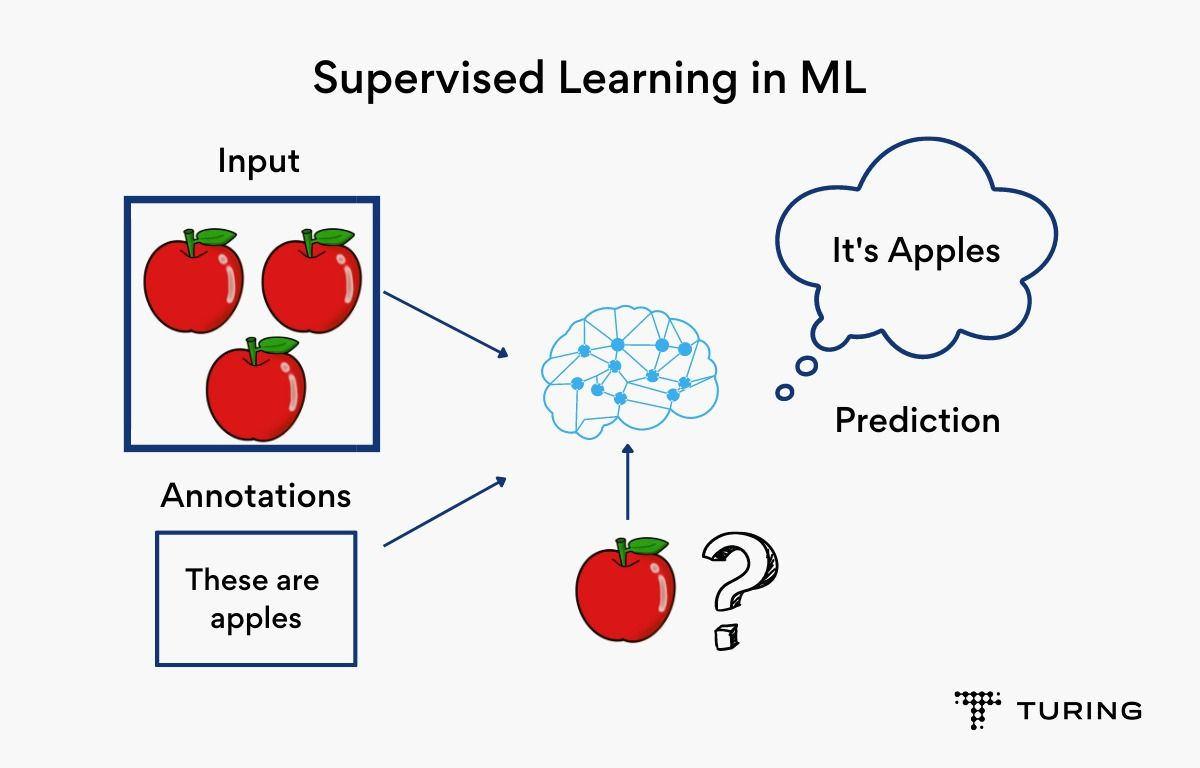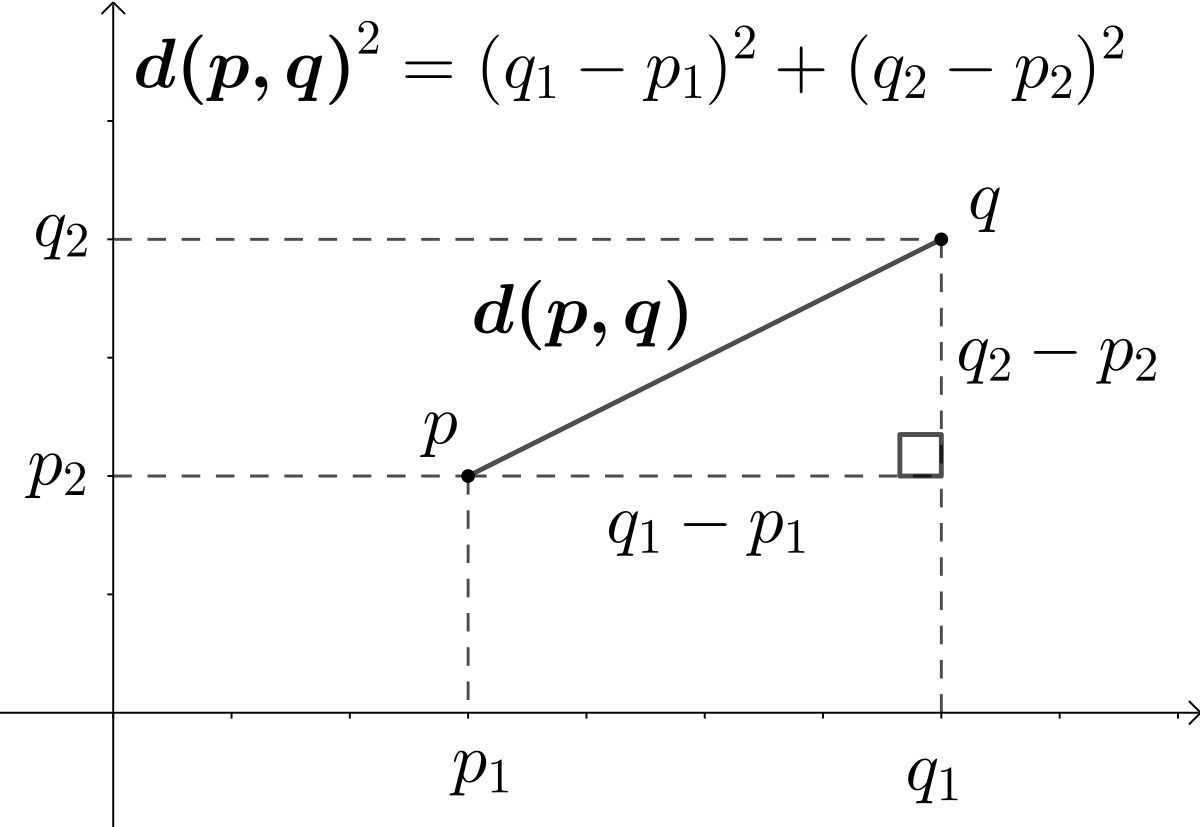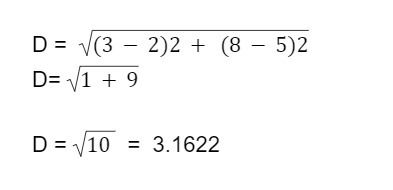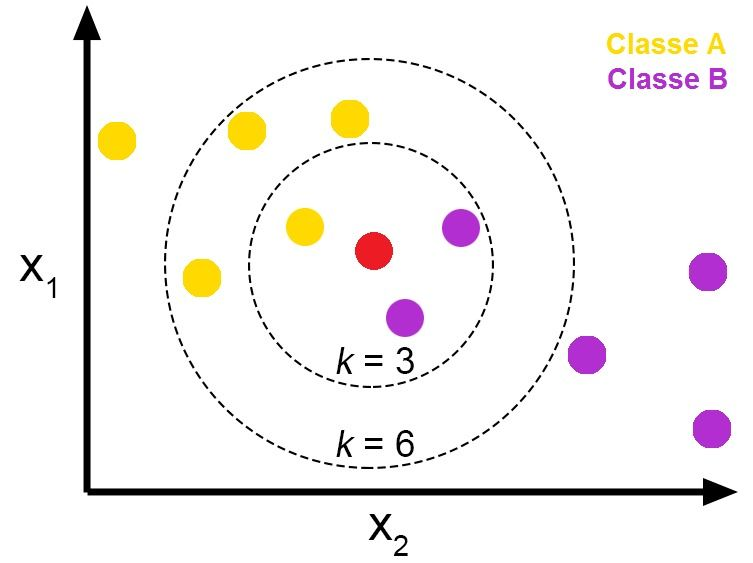# How to Implement KNN Algorithm in PythonKNN algorithm is one of the simplest, yet effective, machine learning algorithms that identifies new data classes based on the existing or available dataset. For example, there are hundreds of TV shows on Netflix, and the genres differ from romance, teen, fantasy, noir, sci-fi, supernatural, anime, and a lot more. However, after watching a series or show, you will find recommended shows and series "because you watched XYZ” on your Netflix home page.

Out of hundreds of TV shows available, Netflix filters some that could be a fit to your choices. This is done with the help of supervised learning. Netflix is able to recommend TV shows for you because of the KNN machine learning algorithms.

In simple terms, KNN recommends a new show based on your previous choices and likings. But how exactly does it work in practice? This article will cover both the theoretical explanation and practical use of the KNN algorithm. Before we discuss this, let's understand supervised learning.

## What is supervised learning?

Supervised learning is a subcategory of machine learning and artificial intelligence. It mainly uses the labeled dataset to train the algorithm that classifies the data or predicts the outcome efficiently. Each labeled dataset belongs to a class. To identify a new, unlabelled dataset, you have to compare it with the existing dataset and assign a class to it.Supervised learning can be related to teaching a child how to identify different colors. We first have to teach the child about different colors and how to differentiate between them: how yellow is different from red and how the color red is not the same as pink. Once trained, you can then ask the child to identify different colors. He/she will easily be able to identify them based on memory.

Supervised learning in much the same way. Instead of a child, a computer takes charge of learning, but the process remains the same. A labeled dataset is known as supervised learning and a set of unlabeled datasets is called unsupervised learning. We use labeled datasets to identify the unlabelled dataset and this requires active human involvement to train this data. This is why it is called "supervised learning”. KNN is a type of supervised algorithm. It is used for both classification and regression problems.

## Understanding KNN algorithm in theory

KNN algorithm classifies new data points based on their closeness to the existing data points. Hence, it is also called K-nearest neighbor algorithm.

For example, if you want to put your house for rent, you will first check the rent prices in your locality. To play fair, you will search for homes with the same appeal and aesthetics as your house in order to compare prices better. The KNN algorithm works similarly.

For a given unknown dataset, the KNN algorithm identifies all the nearest K datasets, compares them with all Ks, and assigns the same label to the most relatable datasets. Finding the similarity requires calculation and is mainly based on the distance between the new dataset and all existing datasets. There are many ways to do that, but mostly, we make use of the Euclidean theorem to calculate the distance between the datasets. It is as follows -Image source

If you have a mystery dataset located at a position (2, 5), and a K dataset at another position (3, 8)

You will find the distance with -The dataset with the shortest distance to the mystery dataset is preferred. Though you do not need to calculate the distance since it's all computational, clarity of the internal process is essential.

Here are the complete steps to implement the KNN algorithm -

1. Assign a number to K and locate all the data points.
2. Compare all K datasets with unknown datasets with the help of the Euclidean theorem.
3. Label the new dataset based on a majority vote.

The K-nearest neighbor algorithm is lazy, meaning it remembers data rather than creating a function from it. Moreover, it does not make assumptions regarding the data - a non-parametric algorithm. The efficiency of the KNN algorithm depends on the proximity of an unknown dataset with that of the existing dataset and how many datasets we compare.

Let's understand how the algorithm's efficiency varies to the value of K.

Choosing the value of K

Choosing the correct value of K is the most critical part of the KNN algorithm as it determines how many neighbors to consider. When the value of K is 1, we call the nearest neighbor algorithm.

To get the proper K value, we have to run the KNN algorithm multiple times and see which one gives the best results with minimum errors. When the value of K is too small, there are chances of noise inflicting data which will not provide quality results. On the other hand, if the value of K is a large number, it becomes computationally complex.Image source

Moreover, when the value of K is small, prediction is less stable. When you slowly increase it, you will see the model give fewer errors because there is more neighbor data to predict the dataset. However, if you see that with the increase in the value of K you encounter a high number of errors, it is time to stop pushing the value higher.

There is no formula to calculate the value of K. However, based on industry-standard, the value is selected by taking a square root of N, where N stands for the total number of samples. Note that this is not a full-proof method. So, take it with a grain of salt as the value of K varies for each problem. What works best is that you try with multiple values of K and see which gives the optimal results for your model.

## Steps to implement KNN algorithm in Python

Following are the steps that need to be implemented in a KNN algorithm in Python -

Step 1: Start by importing Pandas and Sklearn libraries. These are important libraries that can be imported for KNN implementation.

Step 3: Split the dataset. This is part of preprocessing. Attributes and labels are the two major values in which the datasets are split into. The train test split is performed next. Here, you need to divide data into training and testing splits. This will give a better idea about the performance of the algorithm.

Step 4: It’s time to train the model. This involves testing with different values of K and choosing one that gives the least errors. Number 5 is the most commonly used and preferred value of K.

Step 5: The last step is to evaluate the algorithm. However, there is one thing to note here: the value of K keeps changing as per the dataset. For larger datasets, the algorithm may not yield quality results.

It is important to test the model with different values of K. Generally, an odd number is preferred but again, there is no compulsion.

## Pros and cons of using KNN algorithm

### Pros

1. KNN is a simple and intuitive algorithm that works for classification and regression.
2. No explicit training is required. It takes a memory-based approach to predict datasets and adjusts to new datasets instantly.
3. There are different methods users can take to measure the distance between the datasets. Some of them are Euclidean theorem, Minkowski metric, and Manhattan distance. Euclidean theorem is the preferred method.
4. K is the only parameter in the KNN algorithm and that makes the datasets simple to implement.
5. It is widely used for content recommendation. Netflix, Amazon, YouTube, Instagram, and various search engines use it to suggest and accurately predict new content search for users.

### Cons

1. The complexity increases when dealing with large datasets since the complete dataset is predicted each time. There are ways that can help make KNN faster, but it’s still a challenge.
2. Selecting the correct value of K is critical and challenging. If you choose the wrong value for K, the model could become underfit/overfit for the data.
3. The K-nearest neighbor algorithm is computationally inefficient.

KNN algorithm is a machine learning algorithm that determines new datasets based on their proximity to already labeled datasets. KNN is used in many applications including facial, video, and image detection. It is easy to implement KNN because there is no training or excessive use of parameters. But, it is important to note that the value of K should neither be too small nor too large.

The best approach is to slowly increase the value of K and see what works the best. Like all other algorithms, the KNN algorithm has limitations. For instance, it can’t predict rare datasets, like in the case of new diseases. Another drawback of this algorithm is that it is slow and costly because you need plenty of space to store the data. Nonetheless, it is a simple and effective algorithm and can easily resolve many machine learning problems.

## FAQ

1. How does Python implement KNN?

There are 4 steps to implement KNN in Python-

Step 1: Import all the necessary libraries ( Pandas and Numpy ) and load the data. Step 2: Select the new data set and find all the K-neighbors and calculate the distance between them using Euclidean Theorem. Step 3: Predict the nature of the class. Step 4: Check accuracy.

You can test with multiple values of K. But it is better to start with a lesser value and then gradually increase it. The value of K that gives you the least errors is preferable.

2. How to write KNN algorithm?

You start with choosing the right value of the K. Generally, developers choose five or any odd number. For the selected value of K, all the K-nearest neighbors are located, and the distance between the new data point and all the K’s is calculated. The new dataset is labeled the same category of K points that it can most relates to.

3. How KNN algorithm works with example?

Today, the use of KNN algorithms is everywhere. Shopping websites are a prime example. Based on your liking or previous purchases, eCommerce websites, such as Amazon and eBay, sort new products and show them as a recommendation. You can see similar applications on almost every other social media platforms. Youtube and Instagram use this algorithm to recommend users' shorts and Instagram reels based on their previous views and likings.### Press

What's up with Turing? Get the latest news about us here.### Blog